# Area of a Triangle and Equation of a Plane JEE Notes | EduRev

## JEE : Area of a Triangle and Equation of a Plane JEE Notes | EduRev

The document Area of a Triangle and Equation of a Plane JEE Notes | EduRev is a part of the JEE Course Mathematics (Maths) Class 12.
All you need of JEE at this link: JEE

D. AREA OF A TRIANGLE
Show that the area of a triangle whose vertices are the origin and the points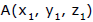and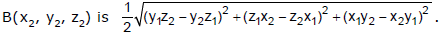The direction ratios of OA are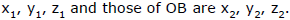Also OA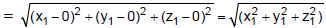and OB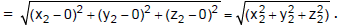∴ the d.c.’ s of OA areand the d.c.’s of OB are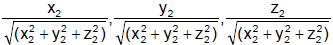Hence if θ is the angle between the line OA and OB, then

sin θ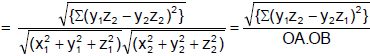Hence the area of ΔOAB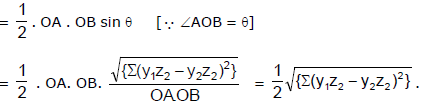Ex.6 Find the area of the triangle whose vertices are A(1, 2, 3), B(2, –1, 1)and C(1, 2, –4).

Sol. Let Δx, Δy, Δz be the areas of the projections of the area Δ of triangle ABC on the yz, zx and xy-planes respectively. We have

Δx =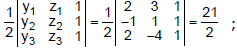Δy =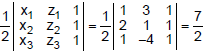Δz =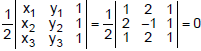∴ the required area Δ =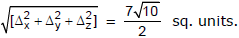Ex.7 A plane is passing through a point P(a, –2a, 2a),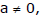at right angle to OP, where O is the origin to meet the axes in A, B and C. Find the area of the triangle ABC.

Sol. OP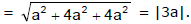Equation of plane passing through P(a, –2a, 2a) is
A(x – a) + B(y + 2a) + C(z – 2a) = 0.
∵ the direction cosines of the normal OP to the plane ABC are proportional to a – 0, –2a – 0, 2a – 0 i.e. a, –2a, 2a. ⇒ equation of plane ABC is

a(x – a) – 2a(y + 2a) + 2a(z – 2a) = 0 or ax – 2ay + 2az = 9a2 ....(1)

Now projection of area of triangle ABC on ZX, XY and YZ planes are the triangles AOC, AOB and BOC respectively.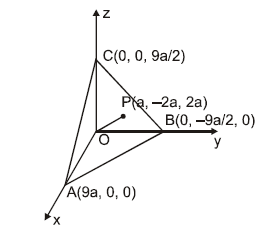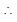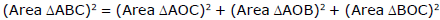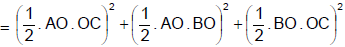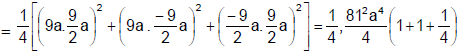Offer running on EduRev: Apply code STAYHOME200 to get INR 200 off on our premium plan EduRev Infinity!

## Mathematics (Maths) Class 12

209 videos|222 docs|124 tests

,

,

,

,

,

,

,

,

,

,

,

,

,

,

,

,

,

,

,

,

,

;